# E due to continous charge distribution

Different types of Continuous Charge Distribution :

Volume Charge Distribution : it is charge distribution spread over a three dimensional volume or region V of space. Volume charge density at any point in this volume as the charge contained per unit volume at that point.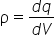the SI unit for ρ is coulomb per cubic meter.If a charge q is distributed over the entire volume of a sphere of radius R, then its volume charge density isThe charge contained in small volume dV is

dq= ρdV

Total electrostatic force exerted on charge q0 due to entire volume V is given by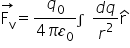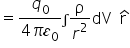electric field due to a volume charge distribution at the location of charge q0 is

It is a charge distribution spread over a two dimensional surface S in space.

Surface charge density at any point on this surface as the charge per unit area at that point.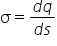The SI unit for σ is coulomb per square meter,

If a charge q is distributed over he surface of a spherical conductor of radius R, then its surface charge density is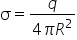The charge contained in small area ds is

dq = σ ds.

Total electrostatic force exerted on charge q0 due to entire surface s is given by

Line charge distribution: It is a charge distribution spread over a one dimensional curve or line L in space. The line charge density at any point on this line as the charge per unit length of the line at that point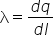The SI unit for σ is coulomb per meter.

If a charge q is distributed over the ring of radius R, then its linear charge density is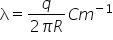The charge contained in small length dl is

dq = λdl

total electrostatic force exerted on charge q0 due to entire length L is given by

Related Keywords